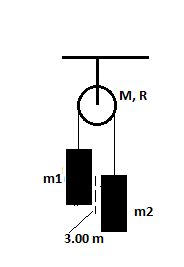# A m_1 = 15.5 kg object and a m_2 = 12.0 kg object are suspended, joined by a cord that passes...

## Question:

A {eq}m_1 {/eq} = 15.5 kg object and a {eq}m_2 {/eq} = 12.0 kg object are suspended, joined by a cord that passes over a pulley with a radius of R = 10.0 cm and a mass M = 3.00 kg. The cord has a negligible mass and does not slip on the pulley. The pulley rotates on its axis without friction. The objects start from rest 3.00 m apart. Treating the pulley as a uniform disk,determine the speeds of the two objects as they pass each other.## Conservation of Energy:

According to energy conservation, the total energy of the system remain constant. Or we can say that the energy changes its form from one to another but the sum of all the energy at any instant is the same.

Given Data:

• Height of the mass 1, {eq}\rm (h_{1}) = 3 \ m {/eq}

Now, the final height of the mass from the reference would be {eq}\rm (h_{2}) = 1.5 \ m {/eq} when they meet.

Therefore, the initial energy of the system would be

{eq}\rm E_{1} = m_{1}gh_{1} \\ E_{1} = 15.5 \times 9.8 \times 3 \\ E_{1} = 455.7 \ J {/eq}

Now, the final energy of the system would be

{eq}\rm E_{2} = (m_{1} + m_{2})gH + \dfrac{1}{2}(m_{1} + m_{2})v^{2} + \dfrac{1}{2}Iw^{2} \\ E_{2} = (m_{1} + m_{2})gH + \dfrac{1}{2}(m_{1} + m_{2})v^{2} + \dfrac{1}{2}\left(\dfrac{MR^{2}}{2}\right)\left(\dfrac{v}{R}\right)^{2} \\ E_{2} = (m_{1} + m_{2})gH + \dfrac{1}{2}(m_{1} + m_{2})v^{2} + \dfrac{1}{4}Mv^{2} \\ E_{2} = [ (15.5 + 12)9.8 \times 1.5] + \dfrac{1}{2}(12+15.5)v^{2} + \dfrac{1}{4}(3)v^{2} \\ E_{2} = 404.25 + 14.5v^{2} \ J {/eq}

Now, applying the energy conservation

{eq}\begin{align} \rm E_{2} &= \rm E_{1} \\ \rm 404.25 + 14.5v^{2} &= 455.7 \\ \rm v &= \rm 1.884 \ m/s \\ \end{align} {/eq}